Performances of Several Univariate Tests of Normality: An Empirical Study | OMICS International
Journal of Biometrics & Biostatistics

Like us on:

# Performances of Several Univariate Tests of Normality: An Empirical Study

Adefisoye JO, Golam Kibria BM* and George F

Department of Mathematics and Statistics, Florida International University, 11200 SW 8th Street, Miami, FL 33199, USA

*Corresponding Author:
Golam Kibria BM
Department of Mathematics and Statistics
Florida International University
11200 SW 8th Street, Miami, FL 33199, USA
Tel: +1 305-348-2000
E-mail: [email protected]

Received Date: September 23, 2016; Accepted Date: November 08, 2016; Published Date: November 11, 2016

Citation: Adefisoye JO, Golam Kibria BM, George F (2016) Performances of Several Univariate Tests of Normality: An Empirical Study. J Biom Biostat 7: 322. doi:10.4172/2155-6180.1000322

Copyright: © 2016 Adefisoye JO, et al. This is an open-access article distributed under the terms of the Creative Commons Attribution License, which permits unrestricted use, distribution, and reproduction in any medium, provided the original author and source are credited.

Visit for more related articles at Journal of Biometrics & Biostatistics

#### Abstract

The problem of testing for normality is fundamental in both theoretical and empirical statistical research. This paper compares the performances of eighteen normality tests available in literature. Since a theoretical comparison is not possible, MonteCarlo simulation were done from various symmetric and asymmetric distributions for different sample sizes ranging from 10 to 1000. The performance of the test statistics are compared based on empirical Type I error rate and power of the test. The simulations results show that the Kurtosis Test is the most powerful for symmetric data and Shapiro Wilk test is the most powerful for asymmetric data.

#### Keywords

Chi-square; Kurtosis; Normality; Shapiro-wilk; Simulation study; Skewness; Type I and type errors

#### AMS Subject Classifications 2010

Primary 62F03, 62F40

#### Introduction

Many of the statistical procedures including correlation, regression, t tests, and analysis of variance are based on the assumption that the data follows a normal distribution or a Gaussian distribution. In many cases, the assumption of normality is critical, when the confidence intervals are developed for population parameters like mean, correlation, variance etc. If the assumptions, under which the statistical procedures are developed, do not hold the conclusion made using these procedures may not be accurate. So, the practitioners need to make sure the assumptions are valid. Checking the validity of the normality assumption in a statistical procedure can be done in two ways: empirical procedure using graphical analysis and the goodnessof- fit tests methods. The goodness-of-fit tests which are formal statistical procedures for assessing the underlying distribution of a data set are our focus here. These tests usually provide more reliable results than graphical analysis. There are many statistical tests available in literature to test whether a given data is from a normal distribution. In this article we review most commonly used methods for normality test and compare them using power and observed significance value.

The first normality test in the literature is the chi-square goodnessof- fit test Snedecor and Cochran  which was suggested by Pearson . Later, the famous Kolmogorov-Smirnov goodness-of-fit test was introduced by Kolmogorov . The Kolmogorov–Smirnov statistic quantifies a distance between the empirical distribution function of the sample and the cumulative distribution function of the reference distribution. The Anderson-Darling test  assesses whether a sample comes from a specified distribution. It makes use of the fact that, when given a hypothesized underlying distribution and assuming the data does arise from this distribution, the frequency of the data can be assumed to follow a Uniform distribution. Lilliefors  test is a modification of Kolmogorov’s test. The Kolmogorov’s test is appropriate when the parameters of the hypothesized normal distribution are completely known whereas in Lilliefors parameters can be estimated from sample. We have included Lilifores test but not KS test in our simulation studies, since in most practical situations we would not know the parameters of null distribution. Shapiro and Wilk  test is the first test that was able to detect departures from normality due to either skewness or kurtosis or both . D’Agostino  proposed a test which is based on transformations of the sample kurtosis and skewness. 0 and Francia  suggested an approximation to the Shapiro-Wilk test which is known to perform well . Jarque- Bera  test is based on the sample skewness and sample kurtosis which uses the Lagrange multiplier procedure on the Pearson family of distributions to obtain tests for normality. In order to improve the efficiency of the Jarque-Bera test, Doornik and Hansen  proposed modification which involves the use of the transformed skewness. The skewness test Bai and Ng  is based on the third sample moment. It is used to test the non-normality due to skewness. In the kurtosis test Bai and Ng [13,14] the coefficient of kurtosis sample data is used to test non-normality due to kurtosis. Gel and Gastwirth proposed a robust modification to the Jarque-Bera test. The Robust Jarque-Bera uses a robust estimate of the dispersion in the skewness and kurtosis instead of the second order central moment. Brys, et al.  have proposed a goodness-of-fit test based on robust measures of skewness and tail weight. Bonett and Seier  have suggested a modified measure of kurtosis for testing normality. Considering that the Brys test is a skewness based test and that the Bonett–Seier is a kurtosis based test a joint test using both these measures was proposed by Romao et al.  for testing normality. The joint test attempts to make use of the two referred focused tests in order to increase the power to detect different kinds of departure from normality. Bontemps and Meddahi  have proposed a family of normality tests based on moment conditions known as Stein equations and their relation with Hermite polynomials. Gel et al.  have proposed a directed normality test, which focuses on detecting heavier tails and outliers of symmetric distributions. Last one in the list is the G test proposed by Chen and Ye .

Over forty (40) different tests have been proposed over time to verify the normality or lack of normality in a population . The main goal of this paper is to compare the performance of most commonly used normality tests in terms of the power of the test and the probability of type I error (α). Yazici and Yolacan  and recently Yap and Sim  did some work on the comparison of normality tests, but kurtosis tests and skewness tests were not in their work. Interestingly, the kurtosis test turned out to be the best test for symmetric distributions and the skewness test performs well for both symmetric and asymmetric distributions.

The rest of the paper is organized as follows. Section 2 discusses different statistical test for normality. A simulation study has been conducted in section 3. A real life data are analyzed in section 4 and finally some concluding remarks are given in section 5.

#### Statistical Methods

There are various parametric and nonparametric tests for normality available in literature. This section discusses widely used statistical methods for normality tests.

Lilliefor’s test [LL]

The test statistic is defined as: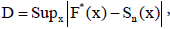Where Sn (x) is the sample cumulative distribution function and F*(x) is the cumulative distribution function (CDF) of the null distribution. For more details and critical values refer Conover .

The AD test is of the form: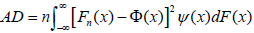Where Fn (x) is the empirical distribution function (EDF), Φ(x) is the cumulative distribution function of the standard normal distribution and ψ(x) is a weight function. The critical values for the Anderson-Darling test along with a more detailed study have been published in Stephens .

Chi-Square test [CS]

The chi-square goodness-of-fit test statistic is defined as: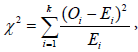where ‘ Oi’ and ‘Ei’ refers to the ith observed and expected frequencies respectively and k is the number of bins/groups. When the null hypothesis is true the above statistic follows a Chi-square distribution with k-1 degrees of freedom.

Skewness test [SK]

The skewness statistic is defined as: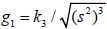, where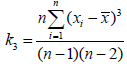and S is the standard deviation.

Under H0, the test statistic Z(g1) is approximately normally distributed for n > 8 and is defined as:,

where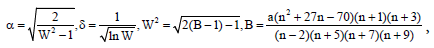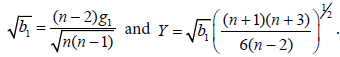Kurtosis test [KU]

The kurtosis statistic is defined as: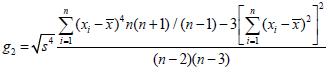Under H0 the test statistic Z(g2) is approximately normally distributed for n ≥ 20 and thus more suitable for this range of sample size. Z(g2) is given as: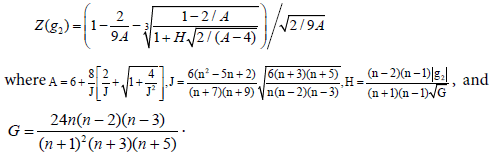#### D’Agostino-Pearson K2 test [DK]

The test combines g1 and g2 to produce an omnibus test of normality. The test statistics is: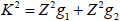Z2 g1 and Z2 g2 are the normal approximations to g1 and g2 respectively. The test statistic follows approximately a chi-square distribution with 2 degree of freedom when a population is normally distributed. The test is appropriate for a sample size of at least twenty.

Shapiro–Wilk test [SW]

The Shapiro-Wilk test uses a W statistic which is defined as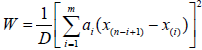Where m=n/2 if n is even while m=(n – 1)/2 if n is odd,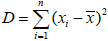and x(i) represents the ith order statistic of the sample, the constants ai are given by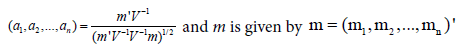Where m1, m2, m1,… mn are the expected values of the order statistics of independent and identically distributed random variables sampled from the standard normal distribution, and V is the covariance matrix of those order statistics. For more information about the Shapiro-Wilk test refer the original Shapiro and Wilk  paper and for critical values refer Pearson and Hartley .

Shapiro-Francia [SF]

The test statistic is defined as: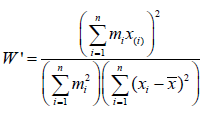The W′ equals the product-moment correlation coefficient between the x(i) and the mi, and therefore measures the straightness of the normal probability plot x(i) ; small values of W′ indicate non-normality. A detailed discussion of this test along with critical values is available in Royston .

Jarque-Bera test [JB]

The test statistic is given as: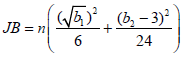Where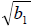and b2 are the skewness and kurtosis measures and are given by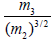and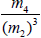respectively; and m2, m3, m4 are the second, third and fourth central moments respectively. The Jarque- Bera statistic is chi-square distributed with two degrees of freedom.

Robust Jarque-Bera test [RJB]

The robust Jarque–Bera (RJB) test statistic is defined as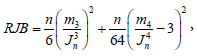Where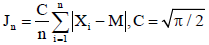and M is the sample median. The RJB statistic is asymptotically χ22-distributed

Doornik-Hansen test [DH]

The test statistic involves the use of the transformed skewness and transformed kurtosis. The transformed skewness is given by the following expression: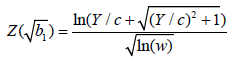where Y, c and w are obtained by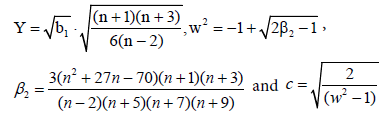Bowman and Shenton  had proposed the transformed kurtosis z2 as follows,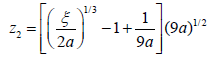With ξ and a obtained by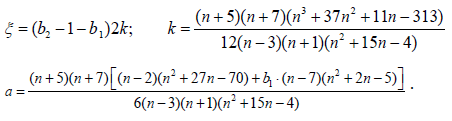The test statistic proposed by Doornik and Hansen  is given by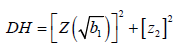The normality hypothesis is rejected for large values of the test statistic. The test is approximately chi-squared distributed with two degrees of freedom.

Brys-Hubert-Struyf MC-MR test [BH]

This test is based on robust measures of skewness and tail weight. The considered robust measure of skewness is the medcouple (MC) defined as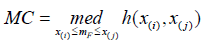where med stands for median mF is the sample median and h is a kernel function given by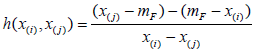The left medcouple (LMC) and the right medcouple (RMC) are the considered robust measures of left and right tail weight respectively and are defined by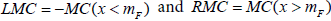The test statistic TMC-LR is then defined by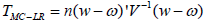in which w is set as [MC, LMC, RMC]’, and ω and V are obtained based on the influence function of the estimators in ω. According to Brys, et al. , for the case of normal distribution, and V are defined respectively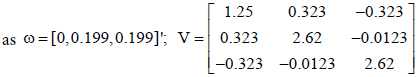The normality hypothesis of the data is rejected for large values of the test statistic which approximately follows the chi-square distribution with three degrees of freedom.

Bonett-Seier test [BS]

The test statistic Tw is given by: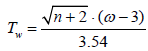in which ω is set by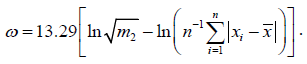This statistic follows a standard normal distribution under null hypothesis.

Brys-Hubert-Struyf-Bonett-Seier Joint test [BHBS]

The normality hypothesis of the data is rejected for the joint test when rejection is obtained for either one of the two individual tests for a significance level of α/2.

Bontemps-Meddahi tests [BM(1) and BM(2)]

The general expression of the test family is given by: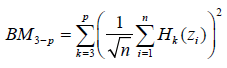Where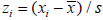and Hk (⋅) represents the kth order normalized Hermite polynomial.

Different tests can be obtained by assigning different values to p, which represents the maximum order of the considered normalized Hermite polynomials in the expression above. Two different tests are considered in this work with p=4 and p=6; these tests are termed BM3-4 and BM3-6. The hypothesis of normality is rejected for large values of the test statistic and according to Bontemps and Meddahi ; the general BM3-p family of tests asymptotically follows the chi-square distribution with p - 2 degree of freedom.

Gel-Miao-Gastwirth test [GMG]

The test is based on the ratio of the standard deviation and on the robust measure of dispersion Jn as defined in the expression: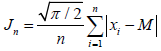where M is the sample median.

The normality test RSJ which should tend to one under a normal distribution is thus given by: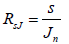The normality hypothesis is rejected for large values of the RSJ, and the statistic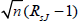is asymptotically normally distributed .

G test [G]

The test is used to test if an underlying population distribution is a uniform distribution. Suppose x1, x2,…, xn are the observations of a random sample from a population distribution with distribution function F(x). Suppose also that x(1), x(2),…, x(n) are the corresponding order statistics. The test statistic has the form: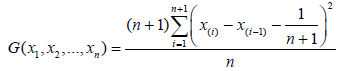Where x(0) is defined as 0, and x(n+1) is defined as 1.

We can observe that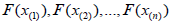are the ordered observations of a random sample from the U(0,1) distribution and thus the G Statistic can be expressed as: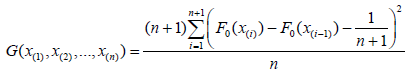When the population distribution is the same as the specified distribution, the value of the test statistic should be close to zero. On the other hand, when the population distribution is far away from the specified distribution, the value should be pretty close to one.

In order to use the test to test for normality, we can assume F(x) to be a normal distribution. Considering the case where the parameters of the distribution are not known, Lilliefor’s idea is adopted by calculating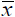and s2 from the sample data and using them as estimates for μ and σ2 respectively, and thus F(x) is the cumulative distribution function of the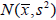distribution. By using the transformation: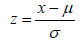The test statistic becomes: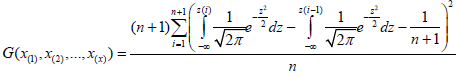The hypothesis of normality should be rejected at significant level α if the test statistic is bigger that it’s 1 - α critical value. A Table 1 of critical values is available in Chen and ye .

Normal (0, 1) – Skewness = 0, Kurtosis = 0
N LL* AD* CS* DK* SK* KU* SW* SF* JB RJB DH* BH BS* BHBS BM(1) BM(2) GMG G*
20 4.67 4.87 4.86 5.8 4.81 4.6 4.68 4.98 2.32 6.03 4.84 1.09 4.67 13.65 2.69 3.6 8.44 4.73
50 4.72 4.87 5.04 5.75 4.9 5.03 4.96 5.17 3.74 5.87 4.81 4.09 4.41 14.02 3.3 6.67 9.05 4.41
100 5.22 5.42 5.01 5.76 4.94 5.47 5.03 5.46 4.49 5.84 5.3 3.76 5.17 14.42 4.23 9.3 10.03 5.55
500 4.77 4.54 5.12 4.83 4.43 5.18 4.56 4.55 4.22 4.17 4.33 4.85 4.83 15.51 4.16 12.23 10.18 4.9

Table 1: Simulated Type I error rate at 5% significance level.

#### Simulation

Since a theoretical comparison among the test statistics is not feasible, a simulation study has been conducted instead to compare the performance of the test statistics in this section.

Simulation techniques

The results of the simulation vary across different levels of significance, sample size and alternative distributions. The results for the 0.05 significance level for the different distribution considered are as presented in the Tables 1-3. First we generate samples of sizes 20, 50, 100 and 500 from standard normal distribution to compare probabilities of type I error. The empirical probability of Type I error is defined as the number of times null hypothesis of normality rejected divided by the total number of simulations. The results in Table 1 are based on 10,000 simulations. We use the software R 3.1.1 R Core Team  for all simulations.

N LL AD CS DK SK KU SW SF JB RJB DH BH BS BHBS BM(1) BM(2) GMG G
Beta (2, 2) – Skewness = 0, Kurtosis = -0.86
20 5.13 6.00 5.26 3.61 0.78 9.48 5.60 2.72 0.11 0.73 2.55 1.46 8.30 14.93* 0.14 0.58 7.99 3.15
50 8.25 13.47 7.35 23.54 0.25 38.86* 15.19 5.62 0.03 0.08 6.10 7.56 21.28 26.97 0.08 10.80 25.41 4.41
100 15.06 31.17 11.25 64.97 0.18 78.79* 44.79 20.82 1.35 0.01 25.31 7.69 46.33 46.10 11.76 47.16 54.35 6.96
500 83.33 99.82 69.55 100.00 0.06 100.00 100.00 100.00 100.00 99.72 100.00 25.03 99.73 99.49 100.00 100.00 99.91 70.53
Beta (3, 3) – Skewness = 0, Kurtosis = -0.67
20 4.56 4.66 4.77 2.58 1.02 5.65 4.11 2.16 0.17 1.11 1.99 1.35 5.74 14.31* 0.08 0.77 6.49 2.80
50 5.88 6.98 5.61 9.80 0.50 18.93 6.59 2.56 0.05 0.20 2.68 5.82 11.36 19.15* 0.03 4.41 14.51 3.42
100 8.16 13.13 7.05 27.73 0.21 42.81* 15.34 5.97 0.23 0.06 7.73 5.98 23.21 28.10 0.13 17.74 29.80 4.39
500 40.98 80.44 26.38 99.26 0.25 99.88* 97.53 90.57 94.20 78.87 96.26 12.81 89.92 87.52 93.88 97.75 94.21 14.16
Uniform (0, 1) – Skewness = 0, Kurtosis = -1.20
20 9.34 16.72 8.09 15.57 0.64 30.12* 19.84 8.14 0.07 0.36 9.31 3.33 21.35 24.14 0.05 1.24 18.77 6.84
50 25.43 56.68 19.57 79.77 0.17 88.59* 74.76 46.87 0.01 0.01 44.40 16.89 61.46 60.62 0.00 58.41 64.67 14.73
100 58.64 94.78 45.61 99.74 0.13 99.90* 99.59 96.74 55.78 4.24 95.06 28.35 93.39 91.45 47.50 98.63 95.17 48.08
500 100.00 100.00 100.00 100.00 0.09 100.00 100.00 100.00 100.00 100.00 100.00 91.55 100.00 100.00 100.00 100.00 100.00 100.00
t(10) – Skewness = 0, Kurtosis = 1
20 7.40 8.75 6.29 12.58 11.07 9.84 9.94 11.63 7.65 14.54 11.97 0.97 8.80 17.07* 8.51 9.87 16.02 6.90
50 8.50 11.59 6.51 18.92 15.23 15.53 14.54 18.72 17.15 22.80 19.01 4.42 14.00 21.55 20.44 22.53 23.35* 6.88
100 11.14 16.35 7.54 27.39 19.78 24.95 23.37 28.61 28.78 33.79 29.17 3.86 21.92 27.86 33.51 37.82* 32.84 7.50
500 28.45 - 12.28 69.96 26.19 74.37 65.55 71.84 75.16 76.42 74.97 6.50 65.98 65.81 80.12 84.05* 75.54 10.72
t (5) – Skewness = 0, Kurtosis = 6
20 12.55 16.87 9.18 23.39 21.03 18.34 18.83 22.57 16.73 26.19* 22.28 0.94 16.71 23.50 14.46 20.44 26.62 4.52
50 21.25 30.39 12.89 41.15 31.92 37.81 36.07 41.96 40.33 47.95* 42.87 4.46 35.79 39.58 39.05 47.00 47.26 7.85
100 33.42 - 18.34 60.04 40.00 59.63 56.37 62.89 62.90 69.02* 64.10 4.47 58.54 59.73 62.22 71.35 68.79 11.61
500 89.27 - 57.90 99.01 56.30 99.41 98.93 99.22 99.38 99.56 99.41 12.21 99.22 99.15 99.37 99.81* 99.60 26.45
Laplace (0, 1) – Skewness = 0, Kurtosis = 3
20 21.40 26.64 14.63 30.23 25.62 23.82 25.59 31.62 22.13 38.28 30.58 1.04 28.34 32.25 18.50 28.11 43.64* 7.46
50 43.15 54.46 28.04 51.89 35.52 49.66 52.77 60.12 51.44 68.84 56.87 5.96 64.55 64.71 49.88 64.29 76.65* 14.46
100 70.97 83.08 47.55 73.76 40.91 76.35 80.08 84.99 78.09 89.49 80.48 10.14 90.25 89.63 77.33 89.05 94.86* 23.09
500 99.99 - 99.42 99.97 50.17 99.99 100.00 100.00 99.99 100.00 99.99 56.45 100.00 100.00 99.99 100.00 100.00 65.01

Table 2: Simulated power for symmetric distributions at 5% significance level.

Gamma (4, 5) – Skewness = 1, Kurtosis = 4
N LL AD CS DK SK KU SW SF JB RJB DH BH BS BHBS BM(1) BM(2) GMG G
20 17.99 25.04 12.80 25.33 29.06 15.23 29.35* 28.72 16.66 25.01 23.36 1.86 8.98 15.93 13.95 22.88 19.18 5.10
50 41.17 59.08 27.29 55.34 67.21 27.16 69.45* 65.96 49.68 53.87 63.88 14.18 14.10 26.52 47.69 65.19 29.40 11.56
100 70.50 89.38 51.49 88.10 94.15 39.31 95.81* 94.38 86.83 84.90 94.74 25.54 17.19 37.93 85.79 94.71 37.72 28.81
500 100.00 - 99.98 100.00 100.00 89.89 100.00 100.00 100.00 100.00 100.00 93.18 40.14 95.54 100.00 100.00 78.55 99.84
Chi-square (3) – Skewness = 1.63, Kurtosis = 4
20 41.37 58.54 41.52 48.03 56.67 27.68 65.81* 62.43 35.86 46.78 54.81 6.68 15.33 22.63 38.74 49.12 35.69 15.01
50 82.14 96.42 84.91 88.63 95.33 52.12 98.87* 98.05 86.37 86.50 97.17 41.66 27.07 56.44 91.32 96.17 57.83 59.96
100 99.11 99.99 99.56 99.97 99.93 75.37 100.00 100.00 99.92 99.71 100.00 75.32 42.01 85.26 100.00 100.00 76.92 98.64
500 100.00 - 100.00 100.00 100.00 99.95 100.00 100.00 100.00 100.00 100.00 100.00 89.71 100.00 100.00 100.00 99.79 100.00
Exponential (1) – Skewness = 2, Kurtosis = 6
20 58.02 77.82 66.21 60.58 70.32 36.38 83.73* 80.15 48.38 59.55 73.27 14.74 20.46 32.04 43.54 65.46 46.95 28.66
50 96.32 99.70 98.44 96.42 98.82 66.25 99.95* 99.84 95.63 95.02 99.63 66.17 38.29 77.95 94.79 99.42 74.15 90.73
100 100.00 100.00 100.00 100.00 100.00 88.86 100.00 100.00 100.00 99.99 100.00 94.51 57.70 97.51 100.00 100.00 91.64 99.99
500 100.00 - 100.00 100.00 100.00 100.00 100.00 100.00 100.00 100.00 100.00 100.00 98.55 100.00 100.00 100.00 100.00 100.00
Log-Normal (0, 1) – Skewness = 6.18, Kurtosis = 113.94
20 78.59 90.30 82.36 79.82 86.91 59.63 93.10* 91.67 71.53 80.43 88.43 21.71 41.48 54.33 67.68 84.36 71.66 52.75
50 99.52 99.99 99.75 99.70 99.91 90.88 100.00 100.00 99.59 99.48 100.00 80.16 77.62 95.05 99.48 99.98 95.52 97.92
100 100.00 - 100.00 100.00 100.00 99.62 100.00 100.00 100.00 100.00 100.00 98.41 96.05 99.87 100.00 100.00 99.81 100.00
500 100.00 - 100.00 100.00 100.00 100.00 100.00 100.00 100.00 100.00 100.00 100.00 100.00 100.00 100.00 100.00 100.00 100.00
Weibull (2, 2) – Skewness = 0.63, Kurtosis = 0.25
20 9.72 12.55 8.01 13.06 14.44 8.64 15.13* 14.10 6.63 12.14 10.41 1.48 5.81 13.24 5.35 9.97 11.04 3.60
50 20.75 31.11 13.71 28.29 37.62 13.10 41.47* 36.02 21.25 24.84 33.37 8.77 7.14 18.10 19.78 35.24 13.78 6.20
100 38.78 60.46 25.45 56.37 69.28 15.57 79.33* 71.64 50.06 47.50 72.64 13.80 8.58 22.05 48.25 72.93 14.79 12.64
500 98.71 100.00 98.30 100.00 100.00 24.71 100.00 100.00 100.00 100.00 100.00 67.76 12.27 69.02 100.00 100.00 15.72 99.07
Gompertz (0.001, 1) – Skewness = -1, Kurtosis = 1.5
20 18.57 26.21 12.68 28.62 31.71* 16.71 30.00 30.45 19.23 28.67 25.44 2.20 9.92 17.49 16.24 25.56 22.06 5.03
50 41.23 57.81 24.89 58.39 68.09* 29.77 66.80 65.27 53.30 58.08 62.51 13.42 16.46 29.14 51.39 65.75 34.79 10.47
100 70.46 87.48 45.79 87.97 94.22* 46.68 93.49 92.40 87.28 86.98 92.56 25.69 23.46 42.58 86.46 93.54 48.16 22.62
500 100.00 100.00 99.90 100.00 100.00 96.22 100.00 100.00 100.00 100.00 100.00 92.55 65.88 97.63 100.00 100.00 92.56 94.33

Table 3: Simulated power for asymmetric distributions at 5% significance level.

The empirical power of a test is calculated as the ratio of the number of times the null hypothesis rejected when the alternative hypothesis of non-normality is true. For power comparison purposes we have considered the following distributions: Beta, Uniform, Student’s t and Laplace and these are class of symmetric distributions. For assymetric class of distributions, we consider Gamma, Chi-square, Exponential, Log-Normal, Weibull and Gompertz distributions. To compare power of the tests we generate samples of sizes 20, 50, 100 and 500 from nonnormal distributions. The power based on 10,000 simulations from different symmetric distributions is presented in Table 2 and those from asymmetric distributions are shown in Table 3. The critical values for corresponding test statistics are discussed in Section 2.

Discussion of simulation results

The best test is the one with maximum power while keeping the nominal significance level. Table 1 gives the type I error rate while Tables 2 and 3 give the power of the tests for the several alternative distributions.

An examination of the performance of the tests in terms of type I error rate shows that the LL, AD, CS, DK, SK, KU, SW, SF, RJB, DH tests were found better than the other tests; these tests have Type I error rates that were around the 5% level specified. The RJB test also have generally acceptable type I error rate but these rate were slightly higher than specified when the sample size was less than 50. The JB, BH, BS, BM (1) and G statistic all have Type I error rates lower than 5% and tend to under-reject while the BHBS, BM (2) and the GMG have Type I error rates higher than 5% and tend to over-reject.

A consideration of the results of power of the tests showed that different tests performed differently under different combinations of the sample size and the significance level. A general and expected pattern was observed that as sample size increase the power of the test also increase.

With Beta (2, 2) and Beta (3, 3) as the alternative distributions, we have symmetric distributions with short tails. With Beta (2, 2), only the KU at 78.79% exhibited significant power when the sample size was less than 100, followed by the CS at 64.97%. However, with the sample size of 200, all the test reached at least 80% except for BHBS at 77.99, SF at 75.40, AD at 70.79% and JB at 61.04%. All other tests do not exhibit significant power especially the SK and BH which had 0.05% and 46.74 % power respectively, even at n=1000, and are clearly not suitable for these conditions. It is noticed that as the value of the parameter increases, the tail of the distribution reduces and consequently the coefficient of kurtosis resulting in a loss of power. In fact, for Beta (3, 3), considerable power was not achieved until when the sample size was 200; the kurtosis test was able to achieve a 79.72% power at this point.

In the case of a Uniform (0, 1) as the alternative distribution, the KU test had a power 88.59% at n=50 to prove being the most powerful under this condition, followed closely by DK (79.77%). With n=100, all tests excepts the LL, CS, SK, JB, RJB, BH, BM (1) and the G had power greater than 80%; the CS, SK, JB, RJB, BH, BM(1) and G particularly proved to be very bad test with n ≤ 50 in this situation with the SK only achieving a power of 0.07% even at n=1000.

For a t(10) (t-distribution with 10 degrees of freedom) distribution, all the test were poor in detecting non-normality; even at n=500, only the BM(1) and BM(2) achieved a power of 80%, followed closely by the RJB (76.42%), GMG (75.54%), JB (75.16%), DH (74.97%), KU (74.37%) and SF (71.84%). All other test had power below 70% at n=500 or less. However, BM (2) is not acceptable as it has unacceptable type I error rate.

For a t(5) distribution that is symmetric and long-tailed, none of the tests was able to achieve a power of 80% even at n=100 with those that achieved closest to this cut-off point being the BM(2) (71.35%), RJB (69.02%), GMG (68.79%), DH (64.10%), JB (62.90%), SF (62.89%) and DK (60.04%).

Considering a Laplace (0, 1) with a mean of zero, the GMG is the most powerful for all sample sizes and achieved a power of 94.86% with n=100, with the AD, SW, SF, RJB, DH, BS, BHBS and BM(2) all achieving power above the 80% threshold. The SK and the G tests are the least powerful under this alternative distribution.

In the situation where the alternative distribution is a Gamma (4, 5), the most powerful test was the SW reaching a power of 95.81% at n=100, it was followed closely by the DH, BM(2), SF, and SK all achieving more than 90% power at n=100. The least powerful under the situation are the G, KU and BS. Both G and KU that did not achieve 80% power until n=500; the BS only achieved a power of 61.99% even at n=1000.

The chi-square (3) distribution proved to be one that was easily identified as being non-normal by all tests with SW(87.19%), SF (83.50%), AD(79.93%) and DH(79.42%) all achieving adequate power even at sample size as small as 30. At n=50, all eighteen tests considered had reached at least the 80% threshold except for the KU, BH, BS, BHBS, GMG and G. The least powerful was the BS test never achieving 100% power at n=1000 whereas all other tests have.

Exponential (1) also proved to be a distribution that was easy for the tests to identify as non-normal with the SW and SF having power above 80% at only n=20. All tests were able to achieve more than 80% power at only n=50 except for the KU, BH, BS, BHBS, and GMG. All tests however surpassed the 80% threshold at n=100 except for the BS which only achieved a 57.70% power at this sample size and proved the least powerful never achieving 100% power at n=1000 whereas all other tests have.

The SW test proved to be the most powerful under the Log-normal alternative distribution achieving a power of 83.73% at n=20, followed closely by its modified form the SF (80.13%). All tests surpassed the 80% threshold at n=40 except for the BH and BS which only achieved power of 65.76% and 69.87% respectively. BHBS, a joint test of the BH and BS however proved more powerful than the individual tests by achieving a power of 89.52 at n=40. However, BHBS is not recommended as it has unacceptable type I error rate.

The result of power on a weibull (2, 2) alternative distribution showed that the SW is the most powerful under this distribution. The test achieved a power of 79.33% at n=100 which is just a little below the 80% rate that is usually described as acceptable. The SW is closely followed by the DH (72.64%) and SF (71.64). The AD, DK, SK, JB, RJB, BM(1) and BM(2) were also able to achieve at least 80% power at n=200. The BS once again proved to be the least powerful among the tests under this distribution by only achieving a power of 16.94%.

An asymmetric, short-tailed Gompertz distribution as an alternative distribution showed the SK test to be powerful, and a strong rival to the popular SW test, however, none of the test was able to achieve 80% power until the sample size was increased to 100 at which point all of the tests except the LL, CS, KU, BH, BS, GMG and G had surpassed the threshold. The BS once more was the least powerful under this distribution; despite most of the tests achieving the 80% threshold and a significant number of them achieving 100% at n=500, the test was only able to achieve 65.88% power.

A weibull (2, 2) distribution also showed RJB as the most powerful for sample sizes of 40 or less and SW for larger sample sizes as against BHBS for a sample size of 10 and SW for larger sample sizes at the 5% level. There is however, the most drastic change in the case of the Gompertz (0.001,1) distribution at 1% level, where the GMG was the most powerful for sample size on 10 and SK for other sample sizes. The SK will probably be the most powerful for a sample size of 10 but for the unavailability of the SK along with the KU and DK for sample sizes less than 20. At the 5% level on the other hand, the RJB was the most powerful for sample sizes of 40 or less and BM (2) for larger sample sizes.

As it is clear from the above discussions that all these tests behave differently depending on the alternative distribution under consideration. Even though the BHBS, BM(2) and GMG showed powerful in certain situations, they are not recommended for testing for normality as they do not effectively control for type I error rate. The results are in good agreement with those obtained in Yap and Sim . A general and expected pattern was observed that as sample size increases the power of the test also increases for all tests.

#### Application

This section highlights the illustration of the performance of the tests using a real life example of medical data. The postmortem interval (PMI) is defined as the elapsed time between death and an autopsy. Knowledge of PMI is considered essential when conducting medical research on human cadavers. The following data (Data Source: Hayes and Lewis ) are PMIs of 22 human brain specimens obtained at autopsy in a recent study:

5.5, 14.5, 6.0, 5.5, 5.3, 5.8, 11.0, 6.1, 7.0, 14.5, 10.4, 4.6, 4.3, 7.2, 10.5, 6.5, 3.3, 7.0, 4.1, 6.2, 10.4, 4.9.

The sample is positively skewed with skewness=0.99 and shorttailed with kurtosis=-0.16,

mean=7.30, SD=3.18 and sample size is 22. The QQ plot of PMI data is given below, which certainly indicates that the data are not symmetric (Figure 1).

The computed values of the test statistics along with their p-values and decisions are presented in Table 4. This dataset was originally modeled by a gamma distribution with shape parameter α=5.25 and scale parameter β=1.39, so one may assume that the hypothesis of normality will be rejected, however, seven of the eighteen test considered failed to reject this hypothesis including the popular DK, SW and SF tests. It can be noted that the coefficient of kurtosis of the data is 0.16 and close enough to that of a normal distribution.

Normality Test Value of test statistic P-value (or Critical Value) Reject Normality or Do not reject at α = 5%
LL 0.2398 0.0019 Reject
CS 15.6364 0.0013 Reject
DK 5.5303 0.063 Do not reject
SK 2.238 0.0252 Reject
KU 0.7222 0.4702 Do not reject
SW 0.9091 0.2378 Do not reject
SF 0.9129 0.2244 Do not reject
JB 4.141 0.1261 Do not reject
RJB 7.9721 0.0186 Reject
DH 8.9722 0.0113 Reject
BH 8.8778 0.031 Reject
BS -0.126 0.8997 Do not reject
BHBS 11.5494 0.021 Reject
BM(1) 3.6023 0.1651 Do not reject
BM(2) 7.5541 0.0229 Reject
GMG 1.0968 0.0439 Reject
G 0.121 -0.0714 Reject

Table 4: Test Results from Postmortem interval data.

#### Summary and Conclusions

We have considered eighteen different tests of normality comprising the most popular along with some of the recently proposed tests. The performance was measured in terms of type I error rate and power of the test [31,32]. The type I error rate is the rate of rejection of the hypothesis of normality for data from the normal distribution while the power of the test is the rate of rejection of normality hypothesis for data generated from a non-normal distribution. We have considered both symmetric and asymmetric distributions in the simulation study. Based on the simulation results we have found several useful test statistics for testing the normality. However, the Kurtosis Test is the most powerful for symmetric data and Shapiro Wilk test  is the most powerful for asymmetric data among all the methods with acceptable type I error rate. The findings of this paper are in good agreement with Yap and Sim , but Kurtosis test and Skewness test were not included in their paper. Interestingly, the kurtosis test turned out to be the best test for symmetric distributions and the Skewness test performs well for both symmetric and asymmetric distributions.

#### Acknowledgements

Authors are thankful to referees for their comments that certainly improved the presentation of the paper.

#### References

Select your language of interest to view the total content in your interested language

### Article Usage

• Total views: 2776
• [From(publication date):
December-2016 - Dec 14, 2019]
• Breakdown by view type
• HTML page views : 2522Can't read the image? click here to refresh